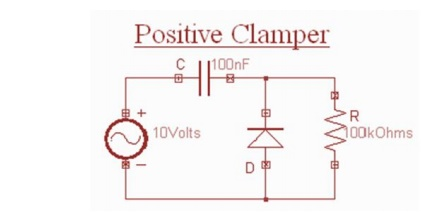Home | | Electronic Circuits II | Positive and Negative Clamper

# Positive and Negative Clamper

The clamping network is one that will ―clamp a signal to a different dc level.

Clamper

The clamping network is one that will ―clamp a signal to a different dc level. The network must have a capacitor, a diode, and a resistive element, but it can also employ an independent dc supply to introduce an additional shift. The magnitude of R and C must be chosen such that the time constant τ= RC is large enough to ensure that the voltage across the capacitor does not discharge significantly during the interval the diode is non conducting.

Throughout the analysis we will assume that for all practical purposes the capacitor will fully charge or discharge in five time constants. The network of Fig. will clamp the input signal to the zero level (for ideal diodes). The resistor R can be the load resistor or a parallel combination of the load resistor and a resistor designed to provide the desired level of R.Diode ―on and the capacitor charging to V volts.

During the interval 0 → T/2 the network will appear, with the diode in the ―on state effectively ―shorting out the effect of the resistor R. The resulting RC time constant is so small (R determined by the inherent resistance of the network) that the capacitor will charge to V volts very quickly. During this interval the output voltage is directly across the short circuit and Vo =0 V. When the input switches to the -V state, the network will appear With an open circuit equivalent for the diode determined by the applied signal and stored voltage across the capacitor—both ―pressuring current through the diode from cathode to anode.

Now that R is back in the network the time constant determined by the RC product is sufficiently large to establish a discharge period much greater than the period T/2 → T, and it can be assumed on an approximate basis that the capacitor holds onto all its charge and, therefore, voltage (since V = Q/C) during this period. Since vo is in parallel with the diode and resistor, it can also be drawn in the alternative position shown in Fig. 2.94. Applying Kirchhoff‘s voltage law around the input loop will result in -V – V – Vo = 0vand Vo =2VDetermining Vo with the diode ―off.Sketching Vo for the network

The negative sign resulting from the fact that the polarity of 2V is opposite to the polarity defined for Vo. The resulting output waveform appears with the input signal. The output signal is clamped to 0 V for the interval 0 to T/2 but maintains the same total swing (2V) as the input. For a clamping network:

The total swing of the output is equal to the total swing of the input signal.

This fact is an excellent checking tool for the result obtained. In general, the following steps may be helpful when analyzing clamping networks:

1. Start the analysis of clamping systems by considering that part of the input signal that will forward bias the diode. The statement above may require skipping an interval of the input signal (as demonstrated in an example to follow), but the analysis will not be extended by an unnecessary measure of investigation.

During the period that the diode is in the ―on state, assume that the capacitor will charge up instantaneously to a voltage level determined by the network.

3.  Assume that during the period when the diode is in the ―off state the capacitor will hold on to its established voltage level.

4.  Throughout the analysis maintain a continual awareness of the location and reference polarity for to ensure that the proper levels for are obtained.

5. Keep in mind the general rule that the total swing of the total output must match the swing of the input signal .

1. Positive Clamper

During the negative half cycle of the input signal, the diode conducts and acts like a short circuit. The output voltage Vo 0 volts . The capacitor is charged to the peak value of input voltage Vm. and it behaves like a battery.During the positive half of the input signal, the diode does not conduct and acts as an open circuit. Hence the output voltage Vo= Vm+ Vm This gives a positively clamped voltage2. Negative Clamper

During the positive half cycle the diode conducts and acts like a short circuit. The capacitor charges to peak value of input voltage Vm. During this interval the output Vo which is taken across the short circuit will be zero During the negative half cycle, the diode is open. The output voltage can be found by applying KVL.Study Material, Lecturing Notes, Assignment, Reference, Wiki description explanation, brief detail
Electronic Circuits : Wave Shaping and Multivibrator Circuits : Positive and Negative Clamper |